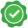##### Get a free home demo of LearnNext

Available for CBSE, ICSE and State Board syllabus.
Call our LearnNext Expert on 1800 419 1234 (tollfree)
OR submit details below for a call back

clear

Rejitha Krishnan RG

Sep 23, 2014

# Prove that its 29th term is double the 19th term.

if 9th term of an AP is zero ,prove that its 29th term is double the 19th term

Ligandro singh

Member since Apr 1, 2017

A9 = 0  Let A be the first term of this Ap and D be the common difference . Therefore A9 = A + (9-1)d (eq  1) = 0         Now A29 = A + 28D and A19 = A + 18D            Now 2 × A19 = 2A + 36 D = A + 28D + A + 8D and we know that A + 8D = 0 from eq 1. Therefore 2 × A19 = A + 28D = A29

Syeda

Answer. In A.P the first term� = a and common difference = d. Given that� 9th term of an A.P. is 0. Therefore t9 = 0 ⇒ a + 8d = 0 ⇒ a = -8d� --------------(1) We have to prove that t29 = 2 t19. t19 = a + 18d = -8d + 18d = 10d������� [� from (1) ] t29� = a + 28d = -8d + 28d = 20d������� [� from (1) ] t29� = 2 x 10d = 2 x t19 ∴ t29� = 2 x t19 .SME Approved

Raghunath Reddy

Sol: In A.P the first term  = a and common difference = d. Given that  9th term of an A.P. is 0. Therefore t9 = 0 ⇒ a + 8d = 0 ⇒ a = -8d  --------------(1) We have to prove that t29 = 2 t19. t19 = a + 18d = -8d + 18d = 10d        [  from (1) ] t29  = a + 28d = -8d + 28d = 20d        [  from (1) ] t29  = 2 x 10d = 2 x t19 ∴ t29  = 2 x t19 .
###### Like NextGurukul? Also explore our advanced self-learning solution LearnNext
Offered for classes 6-12, LearnNext is a popular self-learning solution for students who strive for excellence
Explore
Animated Video
lessons
All India
Test Series
Interactive Video
Experiments
Best-in class
books